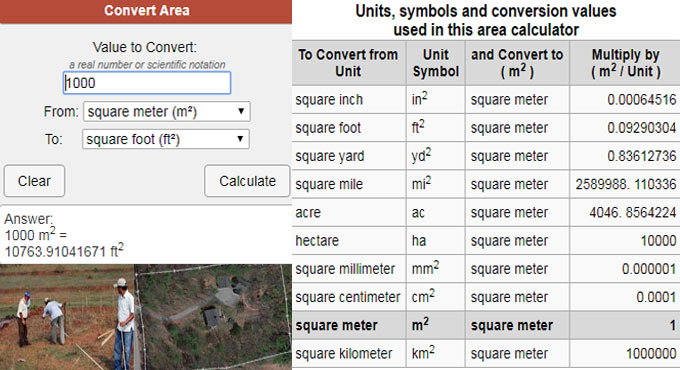# How does area conversion calculator work?Area Conversion Calculator is used to convert units of area by providing the value to be converted to units.

Methods To Convert Units of Area: Conversions are done with the application of a conversion factor. By recognizing the conversion factor, converting among units turns out to be a simple multiplication issue:

S * C = E

Where S denotes the starting value, C denotes the conversion factor, and E denotes end converted result.

To convert from any unit into m2 easily, as for instance, from 50 ft2, just multiply with the value provided in the right column of the table below.

50 ft2 * 0.09290304 [m2 / ft2] = 4.645152 m2

To convert from m2 back into units in the left column divide with the value provided in the right column or, multiply with the reciprocal, 1/x.

4.645152 m2 / 0.09290304 [m2 / ft2] = 50 ft2

To convert between any units in the left column, suppose from A to B, it is necessary to multiply with the factor for A to convert A into m2 then divide by the factor for B to convert out of m2. Or, you can get the single factor as per your requirement by dividing the A factor with the B factor.

As for instance, to convert from ft2 to mm2 you would multiply with 0.09290304 then divide by 0.000001. Or, multiply by 0.09290304/0.000001 = 92903.04. So, to convert directly from ft2 to mm2, it should be multiplied with 92903.04.

To learn how to convert the units, follow this illustration. Suppose, it is required to convert from ft2 to m2; as you can multiply anything with 1 and still retain the original value, but in diverse units, set it up so that ft will cancel out leaving with m.

Since:
1 m = 3.28084 ft, 1 m / 3.28084 ft = 1

The conversion can be written as:

1 ft2 = 1 ft2 (1 m / 3.28084 ft) (1 m / 3.28084 ft) = 0.0929030 m2

And we now have our factor for conversion from ft2 to m2 as 1 * 0.0929030 = 0.0929030. Keep in mind that rounding errors occur in this value. The value in the table, 0.09290304, is more authentic.

Knowing that 1 ft2 = 0.09290304 m2, we can now obtain the conversion factor for converting back. By dividing both sides of the equation with 0.09290304, we get about 10.7639104 ft2 = 1 m2. So, the conversion factor to be multiplied to convert from m2 to ft2 is about 10.7639104.

To perform online calculation, go through the following link calculatorsoup.com